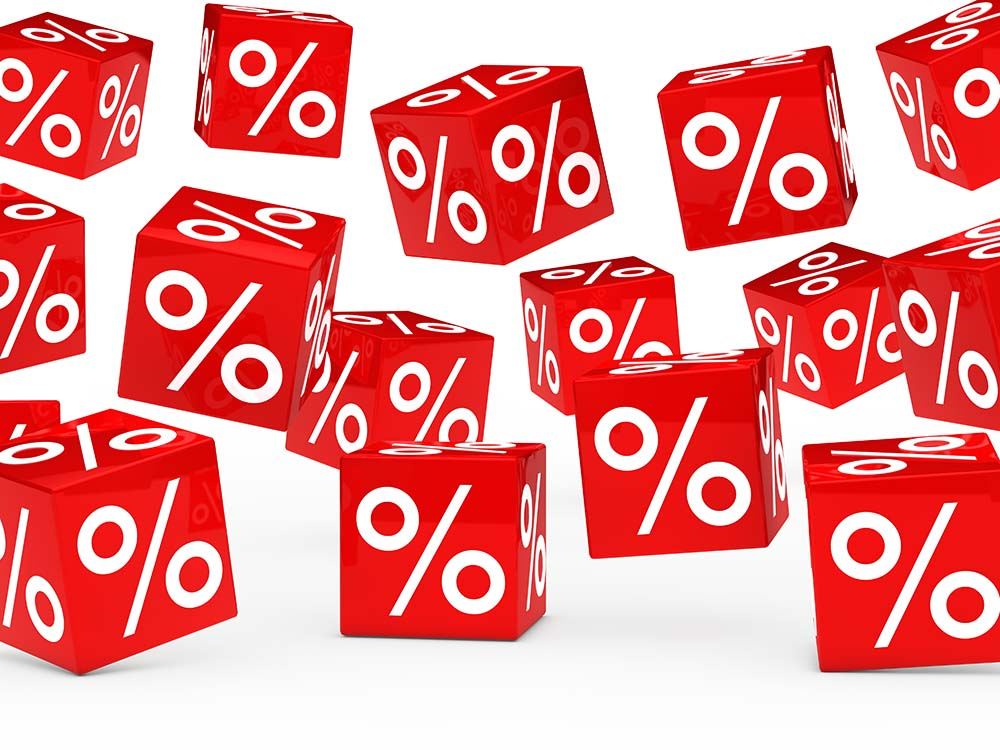Select Page

# How to Calculate the Interest Rate on a LoanAll borrowers know through experience that the cost of a loan depends on the amount borrowed, the rate, and the repayment period, and they see a difference between the nominal rates and the effective rates. But how do you calculate the interest rate on a loan? How does this work?

### Fixed rates and variable rates

Borrowers who want to buy property have the choice between fixed-rate and variable-rate mortgages. While the first option offers the possibility of planning your expenses over the long term and provides a certain peace of mind, the second requires good risk tolerance but usually lets you save money in the long run. There are also loans with interest rates that require a slight tolerance for fluctuations: these are protected variable or “capped” rates.

### Posted rates and real rates

All loans are presented with a nominal rate (also known as the annual contractual rate), but they cost more, because they are actually compounded:

• Semi-annually for mortgages
• Monthly or even daily for other types of loans.
• This has the effect of inflating the effective rate.

For a mortgage compounded semi-annually, a nominal annual rate of 3% is equivalent to an effective annual rate of 3.0225%, applying the formula below:

Real annual rate = (1 + rate per compounding period) – 1.

And because the more often the nominal rate is compounded, the more expensive the loan is, an example with a short-term loan is even more striking: a nominal rate of 24% turns into an effective rate of 26.82% in the case of monthly compounding and 27.11% if the rate is compounded daily.

### The principle of amortization

Apart from payday loans, which are repayable all at once, loans are subject to an amortization schedule calculated based on:

• The amount borrowed
• The repayment period
• The payment frequency
• The interest rate

Each time the borrower makes a payment, they repay a portion of the capital and the interest. And as the payments are made and the amount initially borrowed is reduced, the portion of each payment allocated to the interest decreases, while that allocated to the capital increases.

### A loan calculation example

A borrower who is repaying a \$5,000 loan at a rate of 15% amortized over 48 months will repay \$139.15 per month.

Their first monthly payment will consist of \$76.65 of the principal (or capital repayment) and \$62.50 of the interest payment, and their last monthly payment will consist of \$137.44 of the principal and \$1.72 of the interest payment. In total, the borrower will have repaid \$6,679.38, of which \$1,679.38 is interest.

### A low rate can be deceptive

We can sometimes be tempted by a low rate, thinking that we are paying very little interest. However, the cost of a loan also depends on the amount borrowed and the amortization period. A \$10,000 loan over 72 months therefore still costs \$1,578.43 in interest, to which late payment fees may also be added.

Interest rates on loans are presented to be attractive, hence the importance of knowing the difference between the nominal rate and the effective rate and performing a simulation with the amortization schedule to realize the interest to be paid before taking out a loan.

Click to rate this post!
[Total: 0 Average: 0]